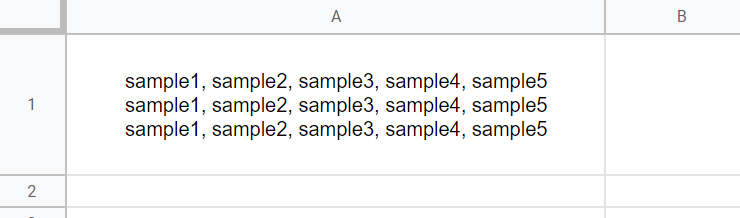# Retrieving Text Positions in Text Data using Google Apps Script

Gists

This is a sample script for retrieving the text positions in the text data using Google Apps Script.

For example, in order to set the rich text style the part of text from the text data, this sample script will be useful.

## Sample situation 1

The sample situation is as follows.

sample1, sample2, sample3, sample4, sample5
sample1, sample2, sample3, sample4, sample5
sample1, sample2, sample3, sample4, sample5


In this sample, the text positions of sample2 and sample5 are retrieved from this sample text data.

### Sample script 1

function myFunction() {
const searchTexts = ["sample2", "sample5"];
const sampleText =
"sample1, sample2, sample3, sample4, sample5\nsample1, sample2, sample3, sample4, sample5\nsample1, sample2, sample3, sample4, sample5\n";

const obj = searchTexts.map((t) => ({
text: t,
position: [...sampleText.matchAll(new RegExp(t, "g"))].map((e) => ({
start: e.index,
end: e.index + e.length,
})),
}));
console.log(obj);
}


When this script is run, the following result is obtained. You can see the text positions of sample2 and sample5 in the sample text can be seen.

[
{
"text": "sample2",
"position": [
{ "start": 9, "end": 16 },
{ "start": 53, "end": 60 },
{ "start": 97, "end": 104 }
]
},
{
"text": "sample5",
"position": [
{ "start": 36, "end": 43 },
{ "start": 80, "end": 87 },
{ "start": 124, "end": 131 }
]
}
]


## Sample situation 2

The sample situation is as follows.

### Input### OutputIn this sample situation, it is found that the text style of sample2 and sample5 of the text in a cell is set to the bold and the red color.

### Sample script 2

#### For one cell

function myFunction() {
const searchTexts = ["sample2", "sample5"];

const range = sheet.getRange("A1");
.setBold(true)
.setForegroundColor("red")
.build();
const richtextValue = range.getRichTextValue();
const sampleText = richtextValue.getText();
const copied = richtextValue.copy();
searchTexts.forEach((t) =>
[...sampleText.matchAll(new RegExp(t, "g"))].forEach((e) =>
copied.setTextStyle(e.index, e.index + e.length, style)
)
);
range.setRichTextValue(copied.build());
}


When this script is run, the value is retrieved from a cell “A1”. And, the text style of sample2 and sample5 is set to the bold and the red color.

#### For multiple cells

function myFunction() {
const searchTexts = ["sample2", "sample5"];

const range = sheet.getDataRange();
.setBold(true)
.setForegroundColor("red")
.build();
const richtextValues = range.getRichTextValues().map((r) =>
r.map((richtextValue) => {
const sampleText = richtextValue.getText();
const copied = richtextValue.copy();
searchTexts.forEach((t) =>
[...sampleText.matchAll(new RegExp(t, "g"))].forEach((e) =>
copied.setTextStyle(e.index, e.index + e.length, style)
)
);
return copied.build();
})
);
range.setRichTextValues(richtextValues);
}


When this script is run, the values are retrieved from the cells of data range. And, the text style of sample2 and sample5 is set to the bold and the red color.

## Updated: May 25 2022

This script was reflected to RichTextApp as a new method ReplaceTextToRichText. By this, you can use this using a Goolge Apps Script library.